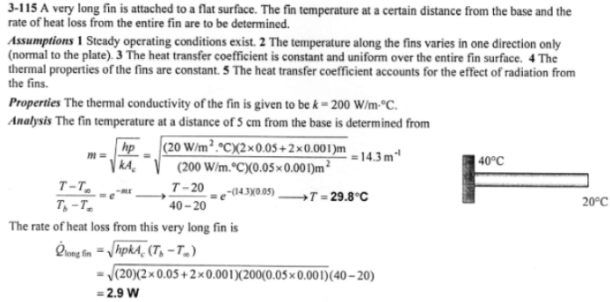heat and mass transfer problems engineering equations heat and mass transfer school homework engineering heat and mass transfer formulas heat and mass transfer solutions to heat and mass transfer problems full solution engineering problem solution heat and mass transfer math problems engineering equations heat and mass transfer school homework engineering solutions to heat and mass transfer formulas heat problems mass problem solutions to transfer problems full solution heat and mass transfer
heat and mass transfer problems engineering equations heat and mass transfer school homework engineering heat and mass transfer formulas heat and mass transfer solutions to heat and mass transfer problems full solution engineering problem solution heat and mass transfer math problems engineering equations heat and mass transfer school homework engineering solutions to heat and mass transfer formulas heat problems mass problem solutions to transfer problems full solution heat and mass transfer
Highalphabet Home Page heat and mass transfer problem solutions Heat and Mass Transfer Page
Consider a very long rectangular fin attached to a flat surface such that the temperature at the end of the fin is essentially that of the surrounding air, i.e. 20 C. Its width is 5.0 cm; thickness is 1.0 mm; thermal conductivity is 200 W/mK; and base temperature is 40 C. The heat transfer coefficient is 20 W/m^2 K. Estimate the fin temperature at a distance of 5.0 cm from the base and the rate of heat loss from the entire fin.Consider a very long rectangular fin attached to a flat surface such that the temperature at the end of the fin is essentially that of the surrounding air, i.e. 20 C. Its width is 5.0 cm; thickness is 1.0 mm; thermal conductivity is 200 W/mK; and base temperature is 40 C. The heat transfer coefficient is 20 W/m^2 K. Estimate the fin temperature at a distance of 5.0 cm from the base and the rate of heat loss from the entire fin.